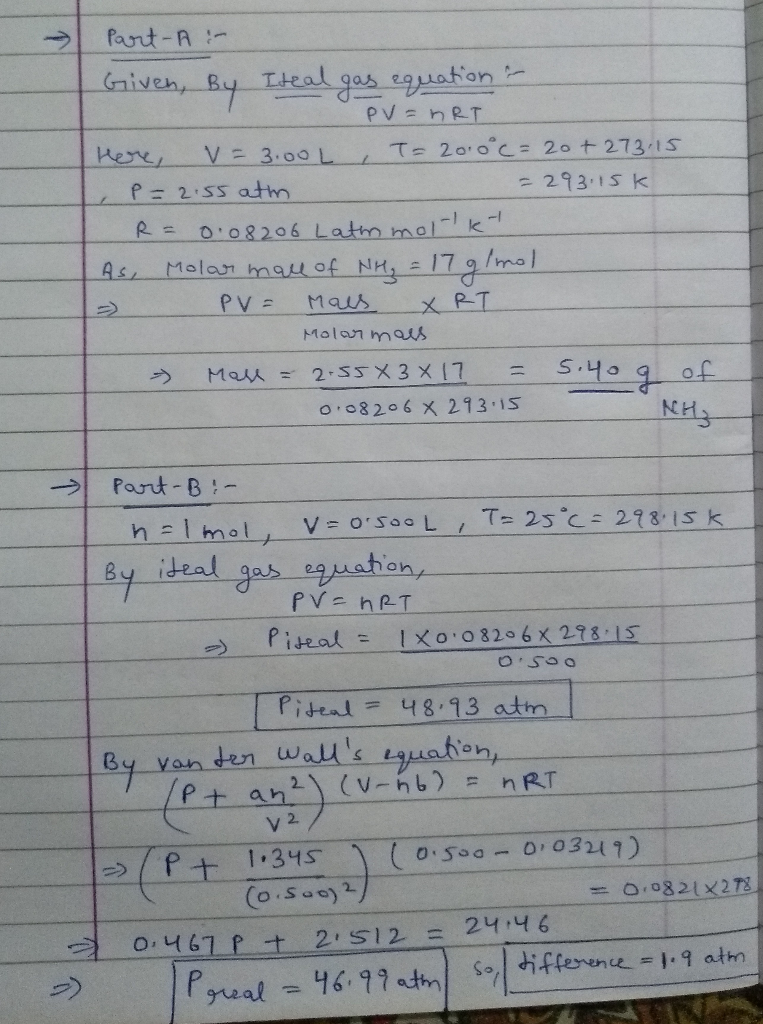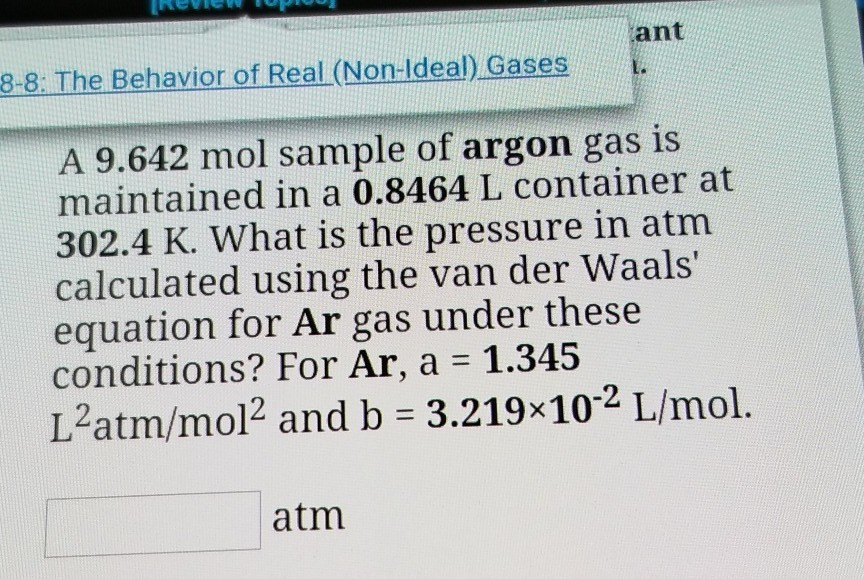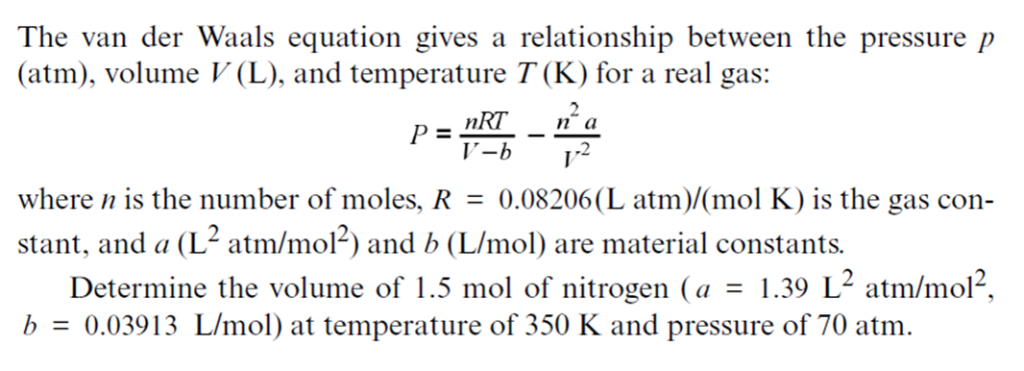Question

# The ideal gas law describes the relationship among the volume of an ideal gas (V), its...

The ideal gas law describes the relationship among the volume of an ideal gas (V), its pressure (P), its absolute temperature (T), and number of moles (n):

PV=nRT

Under standard conditions, the ideal gas law does a good job of approximating these properties for any gas. However, the ideal gas law does not account for all the properties of real gases such as intermolecular attraction and molecular volume, which become more pronounced at low temperatures and high pressures. The van der Waals equation corrects for these factors with the constants a and b, which are unique to each substance:

(P+an2V2)(V−nb)=nRT

The gas constant R is equal to 0.08206 L⋅atm/(K⋅mol).

Part A

A 3.00-L flask is filled with gaseous ammonia, NH3. The gas pressure measured at 20.0 ∘C is 2.55 atm . Assuming ideal gas behavior, how many grams of ammonia are in the flask?

Express your answer to three significant figures and include the appropriate units.

Part B

If 1.00 mol of argon is placed in a 0.500-L container at 25.0 ∘C , what is the difference between the ideal pressure (as predicted by the ideal gas law) and the real pressure (as predicted by the van der Waals equation)? For argon, a=1.345(L2⋅atm)/mol2 and b=0.03219L/mol.

Express your answer to two significant figures and include the appropriate units.#### Earn Coins

Coins can be redeemed for fabulous gifts.

Similar Homework Help Questions
• ### If 1.00 mol of argon is placed in a 0.500-L container at 30.0 ∘C , what...

If 1.00 mol of argon is placed in a 0.500-L container at 30.0 ∘C , what is the difference between the ideal pressure (as predicted by the ideal gas law) and the real pressure (as predicted by the van der Waals equation)? For argon, a=1.345(L2⋅atm)/mol2 and b=0.03219L/mol. Express your answer to two significant figures and include the appropriate units.

• ### If 1.00 mol of argon is placed in a 0.500-L container at 22.0 ∘C , what...

If 1.00 mol of argon is placed in a 0.500-L container at 22.0 ∘C , what is the difference between the ideal pressure (as predicted by the ideal gas law) and the real pressure (as predicted by the van der Waals equation)? For argon, a=1.345(L2⋅atm)/mol2 and b=0.03219L/mol. Express your answer to two significant figures and include the appropriate units.

• ### According to the ideal gas law, a 1.001 mol sample of argon gas in a 1.589...

According to the ideal gas law, a 1.001 mol sample of argon gas in a 1.589 L container at 270.1 K should exert a pressure of 13.96 atm. What is the percent difference between the pressure calculated using the van der Waals' equation and the ideal pressure? For Ar gas, a = 1.345 L2atm/mol2 and b = 3.219×10-2 L/mol.

• ### If 1.00 mol of argon is placed in a 0.500-L container at 30.0 ∘C , what...

If 1.00 mol of argon is placed in a 0.500-L container at 30.0 ∘C , what is the difference between the ideal pressure (as predicted by the ideal gas law) and the real pressure (as predicted by the van der Waals equation)? For argon, a=1.345(L2⋅atm)/mol2 and b=0.03219L/mol.

• ### ant 8-8: The Behavior of Real (Non-Ideal) Gases A 9.642 mol sample of argon gas is...ant 8-8: The Behavior of Real (Non-Ideal) Gases A 9.642 mol sample of argon gas is maintained in a 0.8464 L container at 302.4 K. What is the pressure in atm calculated using the van der Waals' equation for Ar gas under these conditions? For Ar, a = 1.345 L'atm/mol2 and b = 3.219x10-2 L/mol. atm

• ### According to the ideal gas law, a 0.9469 mol sample of argon gas in a 1.474...

According to the ideal gas law, a 0.9469 mol sample of argon gas in a 1.474 L container at 273.1 K should exert a pressure of 14.40 atm. What is the percent difference between the pressure calculated using the van der Waals' equation and the ideal pressure? For Ar gas, a = 1.345 L^2atm/mol^2 and b = 3.219 x 10^-2 L/mol.

• ### According to the ideal gas law, a 9.843 mol sample of argon gas in a 0.8425 L container at 502.0 K should exert a pressure of 481.3 atm

Hint: % difference = 100×(P ideal - Pvan der Waals) / P idealAccording to the ideal gas law, a 9.843 mol sample of argon gas in a 0.8425 L container at 502.0 K should exert a pressure of 481.3 atm. By what percent does the pressure calculated using the van der Waals' equation differ from the ideal pressure? For Ar gas, a =1.345L2 atm/mol2 and b = 3.219×10-2 L/mol.

• ### The van der Waals equation gives a relationship between the pressure p (atm), volume V(L), and temperature T(K) for a real gas: .2 where n is the number of moles, R 0.08206(L atm)(mol K) is the gas c...The van der Waals equation gives a relationship between the pressure p (atm), volume V(L), and temperature T(K) for a real gas: .2 where n is the number of moles, R 0.08206(L atm)(mol K) is the gas con- stant, and a (L- atm/mol-) and b (L/mol) are material constants. Determine the volume of 1.5 mol of nitrogen (a .39 L2 atm/mol2. b = 0.03913 L/mol) at temperature of 350 K and pressure of 70 atm. The van der Waals equation...

• ### According to the ideal gas law, a 1.066 mol sample of krypton gas in a 1.927...

According to the ideal gas law, a 1.066 mol sample of krypton gas in a 1.927 L container at 272.4 K should exert a pressure of 12.37 atm. By what percent does the pressure calculated using the van der Waals' equation differ from the ideal pressure? For Kr gas, a = 2.318 L2atm/mol2 and b = 3.978×10-2 L/mol. ___% Hint: % difference = 100×(P ideal - Pvan der Waals) / P ideal

• ### According to the ideal gas law, a 0.9054 mol sample of krypton gas in a 1.023...

According to the ideal gas law, a 0.9054 mol sample of krypton gas in a 1.023 L container at 274.0 K should exert a pressure of 19.90 atm. What is the percent difference between the pressure calculated using the van der Waals' equation and the ideal pressure? For Kr gas, a = 2.318 L2atm/mol2 and b = 3.978×10-2 L/mol. % According to the ideal gas law, a 9.344 mol sample of oxygen gas in a 0.8267 L container at 500.1...